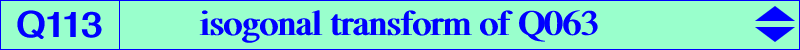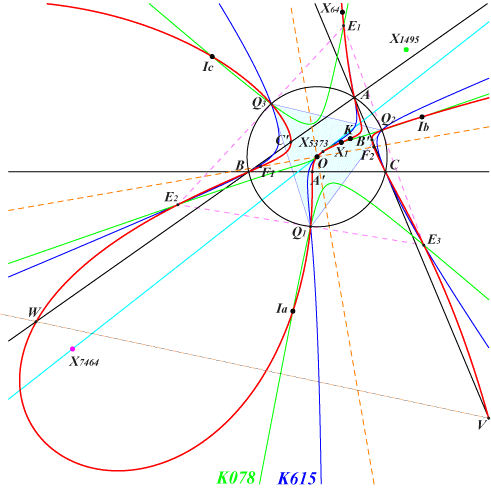too complicated to be written here. Click on the link to download a text file.X(1), X(3), X(6), X(64), X(2574), X(2575), X(5373) excenters of ABC Q1, Q2, Q3 : vertices of the Thomson triangle E1, E2, E3 : excenters of the Thomson triangle circular points at infinity foci of the inconic with center O, perspector X(69) other points belowQ113 is the locus of isopivots Q of isogonal pKs meeting the circumcircle at A, B, C and three other points where the tangents are concurrent. It is the isogonal transform of Q063 which is the locus of the pivots. See CL043. For example, with Q = X(6), X(3), X(64), we obtain the Thomson cubic, the Orthocubic, the Darboux cubic. Q113 is a circular quartic with singular focus X(7464) on the Euler line. It has two real asymptotes parallel at X(1495) to those of the Jerabek hyperbola. The tangents at Q1, Q2, Q3 concur at X(3) and those at the in/excenters of ABC concur at X(6). Q113 meets the sidelines of ABC again at the vertices A', B', C' of the cevian triangle of X(5374) and the traces U, V, W of the trilinear polar of this same point. Q113 meets the Brocard axis at X(3), X(6) and two other points on the rectangular circum-hyperbola with perspector X(2492) passing through X(23), X(316), X(842), etc. Q113 meets the line OI at X(1), X(3) and two other points on the circum-conic passing through X(19), X(269), X(513), X(516), etc. Under extraversion, six other points are obtained on the lines passing through O and the excenters of ABC.